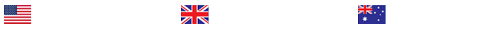Logarithmic Functions

Logarithmic FunctionsWorksheet that goes along with a gizmo found at explorelearning.comA logarithmic function is the inverse of an exponential function. When the Gizmotm starts, you will see the graph of a logarithmic function, y = loga(x), and the graph of its associated exponential function, y = ax.• With Show associated exponential turned on, use the slider to vary the value of a. In the logarithmic function and in the exponential function, a is called the base.• How does the graph of the logarithmic function compare to the graph of the associated exponential function?• How do changes in the base affect the graph of the exponential function?• How do changes in the base affect the graph of the logarithmic function?• Turn on Show line y = x and vary the value of a. How would you describe the relationship between the logarithmic function, the exponential function, and the line y = x?• If a point (C1, C2) is part of a function, then the point (C2, C1) is part of the inverse of the function. With Show associated exponential turned on, set a = 5 to graph the logarithmic function y = log5(x) and the exponential function y = 5x. (To quickly set a slider to a specific number, type the number into the field to the right of the slider, and then press ENTER.)• Turn on Show probe and drag the probe to find the point (5, 1) on the graph of the logarithmic function. What point must exist on the graph of the associated exponential function? Verify your answer by locating this point on the graph of the exponential function.• What is the x-intercept of the logarithmic function? Use the probe to find this value on the graph. How does this intercept relate to the associated exponential function• Vary the value of a. In general, what is the x-intercept of the logarithmic function? How does this intercept relate to the associated exponential function?• Does the exponential function y = ax have an x-intercept? Does the logarithmic function y = loga(x) have a y-intercept? Why or why not?• Graph the function y = log3(x) by setting a = 3. Turn on Show probe, and drag the probe to x = 1.• What is the output, or y-value, of this function when x = 1?• Vary the value of a and observe the output of the function. What do you notice about the output when x = 1?• What can you conclude about the x-intercept of a logarithmic function y = loga(x)?• Based on this result, what can you conclude about the y-intercept of the associated exponential function y = ax? Verify this result by turning on Show associated exponential and examining the graph of y = ax as you vary the value of a.• Graph the function y = log2(x) by setting a = 2. Turn on Show probe, and drag the probe to see the output of this function for different values of x.• What is the output of this function when x = 2, when x = 4, and when x = 8?• Based on these output values, what do you think the output will be when x = 16? Use the probe to check your answer.• How would you describe the meaning of loga(x)?• Is it possible for a to equal 1 in the logarithmic function y = loga(x)? Would the function y = log1(x) have any meaning for an input like x = 4? Why or why not?

### Last Completed Projects

# topic title discipline academic level pages delivered
6
Writer's choice
University
2
1 hour 32 min
7
Wise Approach to
Philosophy
College
2
2 hours 19 min
8
1980's and 1990
History
College
3
2 hours 20 min
9
pick the best topic
Finance
School
2
2 hours 27 min
10
finance for leisure
Finance
University
12
2 hours 36 min